# sklearn.gaussian_process.kernels.RBF¶

class sklearn.gaussian_process.kernels.RBF(length_scale=1.0, length_scale_bounds=(1e-05, 100000.0))[source]

Radial basis function kernel (aka squared-exponential kernel).

The RBF kernel is a stationary kernel. It is also known as the “squared exponential” kernel. It is parameterized by a length scale parameter $$l>0$$, which can either be a scalar (isotropic variant of the kernel) or a vector with the same number of dimensions as the inputs X (anisotropic variant of the kernel). The kernel is given by:

$k(x_i, x_j) = \exp\left(- \frac{d(x_i, x_j)^2}{2l^2} \right)$

where $$l$$ is the length scale of the kernel and $$d(\cdot,\cdot)$$ is the Euclidean distance. For advice on how to set the length scale parameter, see e.g. .

This kernel is infinitely differentiable, which implies that GPs with this kernel as covariance function have mean square derivatives of all orders, and are thus very smooth. See , Chapter 4, Section 4.2, for further details of the RBF kernel.

Read more in the User Guide.

New in version 0.18.

Parameters:
length_scalefloat or ndarray of shape (n_features,), default=1.0

The length scale of the kernel. If a float, an isotropic kernel is used. If an array, an anisotropic kernel is used where each dimension of l defines the length-scale of the respective feature dimension.

length_scale_boundspair of floats >= 0 or “fixed”, default=(1e-5, 1e5)

The lower and upper bound on ‘length_scale’. If set to “fixed”, ‘length_scale’ cannot be changed during hyperparameter tuning.

Attributes:
anisotropic
bounds

Returns the log-transformed bounds on the theta.

hyperparameter_length_scale
hyperparameters

Returns a list of all hyperparameter specifications.

n_dims

Returns the number of non-fixed hyperparameters of the kernel.

requires_vector_input

Returns whether the kernel is defined on fixed-length feature vectors or generic objects.

theta

Returns the (flattened, log-transformed) non-fixed hyperparameters.

References

Examples

>>> from sklearn.datasets import load_iris
>>> from sklearn.gaussian_process import GaussianProcessClassifier
>>> from sklearn.gaussian_process.kernels import RBF
>>> kernel = 1.0 * RBF(1.0)
>>> gpc = GaussianProcessClassifier(kernel=kernel,
...         random_state=0).fit(X, y)
>>> gpc.score(X, y)
0.9866...
>>> gpc.predict_proba(X[:2,:])
array([[0.8354..., 0.03228..., 0.1322...],
[0.7906..., 0.0652..., 0.1441...]])


Methods

 __call__(X[, Y, eval_gradient]) Return the kernel k(X, Y) and optionally its gradient. clone_with_theta(theta) Returns a clone of self with given hyperparameters theta. Returns the diagonal of the kernel k(X, X). get_params([deep]) Get parameters of this kernel. Returns whether the kernel is stationary. set_params(**params) Set the parameters of this kernel.

Return the kernel k(X, Y) and optionally its gradient.

Parameters:
Xndarray of shape (n_samples_X, n_features)

Left argument of the returned kernel k(X, Y)

Yndarray of shape (n_samples_Y, n_features), default=None

Right argument of the returned kernel k(X, Y). If None, k(X, X) if evaluated instead.

Determines whether the gradient with respect to the log of the kernel hyperparameter is computed. Only supported when Y is None.

Returns:
Kndarray of shape (n_samples_X, n_samples_Y)

Kernel k(X, Y)

K_gradientndarray of shape (n_samples_X, n_samples_X, n_dims), optional

The gradient of the kernel k(X, X) with respect to the log of the hyperparameter of the kernel. Only returned when eval_gradient is True.

property bounds

Returns the log-transformed bounds on the theta.

Returns:
boundsndarray of shape (n_dims, 2)

The log-transformed bounds on the kernel’s hyperparameters theta

clone_with_theta(theta)[source]

Returns a clone of self with given hyperparameters theta.

Parameters:
thetandarray of shape (n_dims,)

The hyperparameters

diag(X)[source]

Returns the diagonal of the kernel k(X, X).

The result of this method is identical to np.diag(self(X)); however, it can be evaluated more efficiently since only the diagonal is evaluated.

Parameters:
Xndarray of shape (n_samples_X, n_features)

Left argument of the returned kernel k(X, Y)

Returns:
K_diagndarray of shape (n_samples_X,)

Diagonal of kernel k(X, X)

get_params(deep=True)[source]

Get parameters of this kernel.

Parameters:
deepbool, default=True

If True, will return the parameters for this estimator and contained subobjects that are estimators.

Returns:
paramsdict

Parameter names mapped to their values.

property hyperparameters

Returns a list of all hyperparameter specifications.

is_stationary()[source]

Returns whether the kernel is stationary.

property n_dims

Returns the number of non-fixed hyperparameters of the kernel.

property requires_vector_input

Returns whether the kernel is defined on fixed-length feature vectors or generic objects. Defaults to True for backward compatibility.

set_params(**params)[source]

Set the parameters of this kernel.

The method works on simple kernels as well as on nested kernels. The latter have parameters of the form <component>__<parameter> so that it’s possible to update each component of a nested object.

Returns:
self
property theta

Returns the (flattened, log-transformed) non-fixed hyperparameters.

Note that theta are typically the log-transformed values of the kernel’s hyperparameters as this representation of the search space is more amenable for hyperparameter search, as hyperparameters like length-scales naturally live on a log-scale.

Returns:
thetandarray of shape (n_dims,)

The non-fixed, log-transformed hyperparameters of the kernel

## Examples using sklearn.gaussian_process.kernels.RBF¶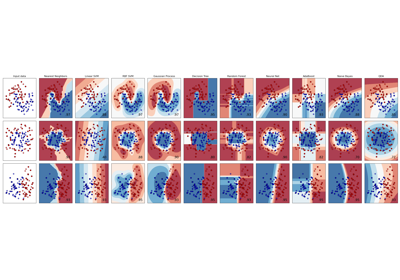Classifier comparison

Classifier comparison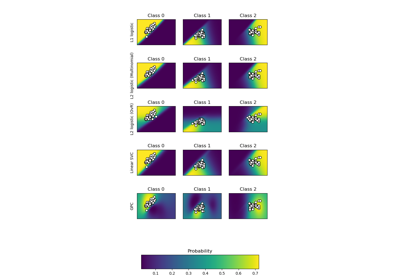Plot classification probability

Plot classification probability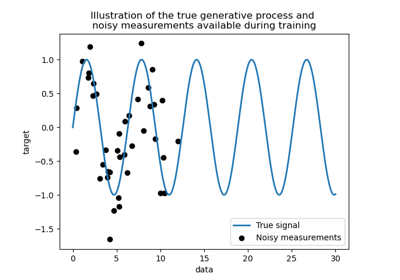Comparison of kernel ridge and Gaussian process regression

Comparison of kernel ridge and Gaussian process regressionGaussian Processes regression: basic introductory example

Gaussian Processes regression: basic introductory example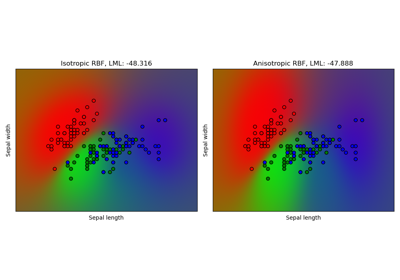Gaussian process classification (GPC) on iris dataset

Gaussian process classification (GPC) on iris dataset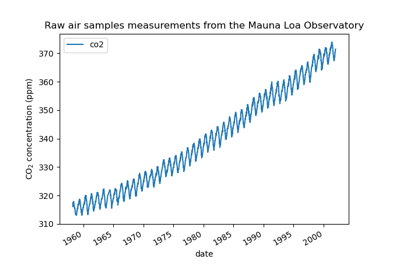Gaussian process regression (GPR) on Mauna Loa CO2 data

Gaussian process regression (GPR) on Mauna Loa CO2 data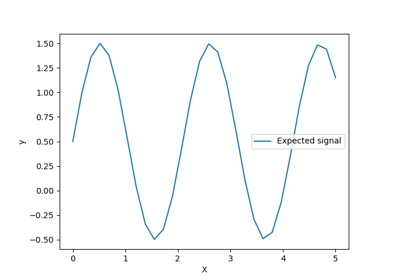Gaussian process regression (GPR) with noise-level estimation

Gaussian process regression (GPR) with noise-level estimation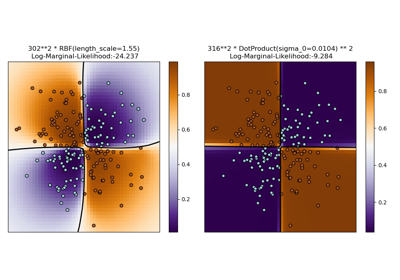Illustration of Gaussian process classification (GPC) on the XOR dataset

Illustration of Gaussian process classification (GPC) on the XOR dataset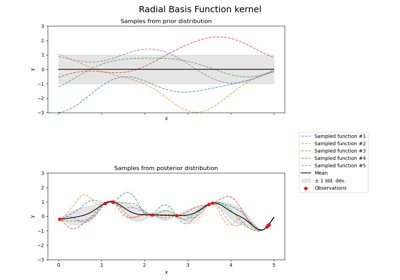Illustration of prior and posterior Gaussian process for different kernels

Illustration of prior and posterior Gaussian process for different kernels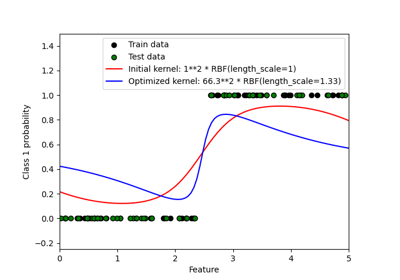Probabilistic predictions with Gaussian process classification (GPC)

Probabilistic predictions with Gaussian process classification (GPC)